### MEMO 2011 ekipno problem 6

Kvaliteta:
Avg: 2,0
Težina:
Avg: 6,0
Let$ABC$ be an acute triangle. Denote by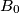$B_0$ and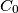$C_0$ the feet of the altitudes from vertices$B$ and$C$, respectively. Let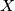$X$ be a point inside the triangle$ABC$ such that the line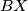$BX$ is tangent to the circumcircle of the triangle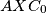$AXC_0$ and the line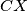$CX$ is tangent to the circumcircle of the triangle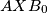$AXB_0$. Show that the line$AX$ is perpendicular to$BC$.
Izvor: Srednjoeuropska matematička olimpijada 2011, ekipno natjecanje, problem 6